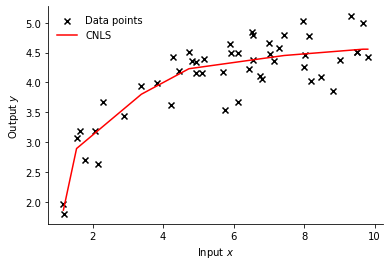# Welcome to pyStoNED¶

pyStoNED is a Python package that provides functions for estimating Convex Nonparametric Least Square (CNLS), Stochastic Nonparametric Envelopment of Data (StoNED), and other various StoNED-related variants such as Convex Quantile Regression (CQR), Convex Expectile Regression (CER), and Isotonic CNLS (ICNLS). It also provides efficiency measurement using Data Envelopement Analysis (DEA) and Free Disposal Hull (FDH). The pyStoNED package allows the user to estimate the CNLS/StoNED frontiers in an open-access environment and is built based on the Pyomo.

For example [.ipynb], the following code estimates the basic CNLS model and plot the production frontier. 

```# import packages
import numpy as np
from pystoned import CNLS
from pystoned.plot import plot2d
from pystoned.constant import CET_ADDI, FUN_PROD, RTS_VRS

# set seed
np.random.seed(0)

# generate DMUs: DGP
x = np.sort(np.random.uniform(low=1, high=10, size=50))
u = np.abs(np.random.normal(loc=0, scale=0.7, size=50))
y_true = 3 + np.log(x)
y = y_true - u

# define the CNLS model
model = CNLS.CNLS(y, x, z=None, cet = CET_ADDI, fun = FUN_PROD, rts = RTS_VRS)
# solve the model with remote solver